## Moving Average Aggregationedit

[6.4.0] Deprecated in 6.4.0. The Moving Average aggregation has been deprecated in favor of the more general Moving Function Aggregation. The new Moving Function aggregation provides all the same functionality as the Moving Average aggregation, but also provides more flexibility.

Given an ordered series of data, the Moving Average aggregation will slide a window across the data and emit the average value of that window. For example, given the data `[1, 2, 3, 4, 5, 6, 7, 8, 9, 10]`, we can calculate a simple moving average with windows size of `5` as follows:

• (1 + 2 + 3 + 4 + 5) / 5 = 3
• (2 + 3 + 4 + 5 + 6) / 5 = 4
• (3 + 4 + 5 + 6 + 7) / 5 = 5
• etc

Moving averages are a simple method to smooth sequential data. Moving averages are typically applied to time-based data, such as stock prices or server metrics. The smoothing can be used to eliminate high frequency fluctuations or random noise, which allows the lower frequency trends to be more easily visualized, such as seasonality.

### Syntaxedit

A `moving_avg` aggregation looks like this in isolation:

```{
"moving_avg": {
"buckets_path": "the_sum",
"model": "holt",
"window": 5,
"gap_policy": "insert_zeros",
"settings": {
"alpha": 0.8
}
}
}```

Table 15. `moving_avg` Parameters

 Parameter Name Description Required Default Value `buckets_path` Path to the metric of interest (see `buckets_path` Syntax for more details Required `model` The moving average weighting model that we wish to use Optional `simple` `gap_policy` Determines what should happen when a gap in the data is encountered. Optional `insert_zeros` `window` The size of window to "slide" across the histogram. Optional `5` `minimize` If the model should be algorithmically minimized. See Minimization for more details Optional `false` for most models `settings` Model-specific settings, contents which differ depending on the model specified. Optional

`moving_avg` aggregations must be embedded inside of a `histogram` or `date_histogram` aggregation. They can be embedded like any other metric aggregation:

```POST /_search
{
"size": 0,
"aggs": {
"my_date_histo":{
"date_histogram":{
"field":"date",
"calendar_interval":"1M"
},
"aggs":{
"the_sum":{
"sum":{ "field": "price" }
},
"the_movavg":{
"moving_avg":{ "buckets_path": "the_sum" }
}
}
}
}
}```
 A `date_histogram` named "my_date_histo" is constructed on the "timestamp" field, with one-day intervals A `sum` metric is used to calculate the sum of a field. This could be any metric (sum, min, max, etc) Finally, we specify a `moving_avg` aggregation which uses "the_sum" metric as its input.

Moving averages are built by first specifying a `histogram` or `date_histogram` over a field. You can then optionally add normal metrics, such as a `sum`, inside of that histogram. Finally, the `moving_avg` is embedded inside the histogram. The `buckets_path` parameter is then used to "point" at one of the sibling metrics inside of the histogram (see `buckets_path` Syntax for a description of the syntax for `buckets_path`.

An example response from the above aggregation may look like:

```{
"took": 11,
"timed_out": false,
"_shards": ...,
"hits": ...,
"aggregations": {
"my_date_histo": {
"buckets": [
{
"key_as_string": "2015/01/01 00:00:00",
"key": 1420070400000,
"doc_count": 3,
"the_sum": {
"value": 550.0
}
},
{
"key_as_string": "2015/02/01 00:00:00",
"key": 1422748800000,
"doc_count": 2,
"the_sum": {
"value": 60.0
},
"the_movavg": {
"value": 550.0
}
},
{
"key_as_string": "2015/03/01 00:00:00",
"key": 1425168000000,
"doc_count": 2,
"the_sum": {
"value": 375.0
},
"the_movavg": {
"value": 305.0
}
}
]
}
}
}```

### Modelsedit

The `moving_avg` aggregation includes four different moving average "models". The main difference is how the values in the window are weighted. As data-points become "older" in the window, they may be weighted differently. This will affect the final average for that window.

Models are specified using the `model` parameter. Some models may have optional configurations which are specified inside the `settings` parameter.

#### Simpleedit

The `simple` model calculates the sum of all values in the window, then divides by the size of the window. It is effectively a simple arithmetic mean of the window. The simple model does not perform any time-dependent weighting, which means the values from a `simple` moving average tend to "lag" behind the real data.

```POST /_search
{
"size": 0,
"aggs": {
"my_date_histo":{
"date_histogram":{
"field":"date",
"calendar_interval":"1M"
},
"aggs":{
"the_sum":{
"sum":{ "field": "price" }
},
"the_movavg":{
"moving_avg":{
"buckets_path": "the_sum",
"window" : 30,
"model" : "simple"
}
}
}
}
}
}```

A `simple` model has no special settings to configure

The window size can change the behavior of the moving average. For example, a small window (`"window": 10`) will closely track the data and only smooth out small scale fluctuations: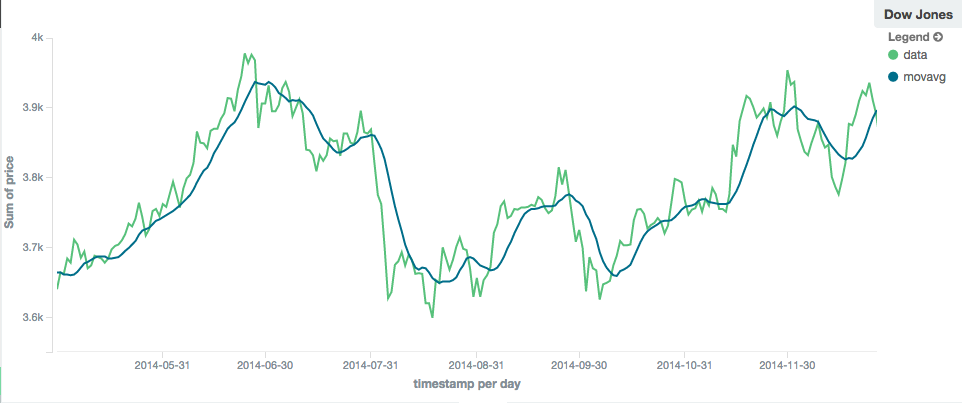Figure 1. Moving average with window of size 10

In contrast, a `simple` moving average with larger window (`"window": 100`) will smooth out all higher-frequency fluctuations, leaving only low-frequency, long term trends. It also tends to "lag" behind the actual data by a substantial amount: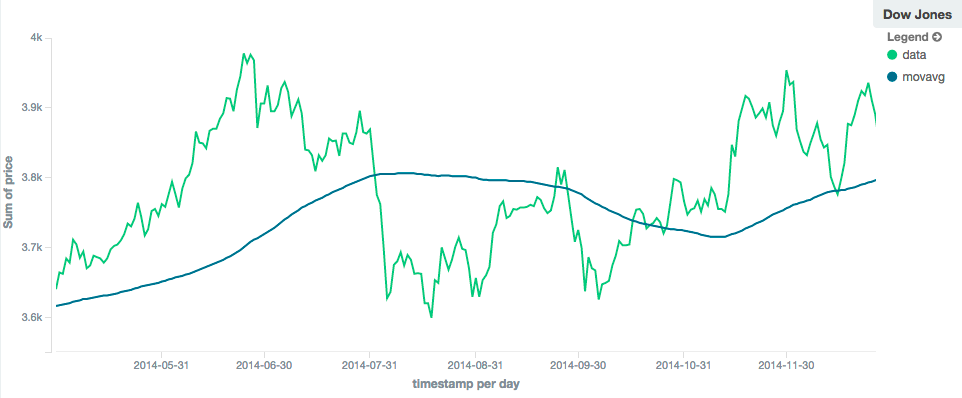Figure 2. Moving average with window of size 100

### Linearedit

The `linear` model assigns a linear weighting to points in the series, such that "older" datapoints (e.g. those at the beginning of the window) contribute a linearly less amount to the total average. The linear weighting helps reduce the "lag" behind the data’s mean, since older points have less influence.

```POST /_search
{
"size": 0,
"aggs": {
"my_date_histo":{
"date_histogram":{
"field":"date",
"calendar_interval":"1M"
},
"aggs":{
"the_sum":{
"sum":{ "field": "price" }
},
"the_movavg": {
"moving_avg":{
"buckets_path": "the_sum",
"window" : 30,
"model" : "linear"
}
}
}
}
}
}```

A `linear` model has no special settings to configure

Like the `simple` model, window size can change the behavior of the moving average. For example, a small window (`"window": 10`) will closely track the data and only smooth out small scale fluctuations: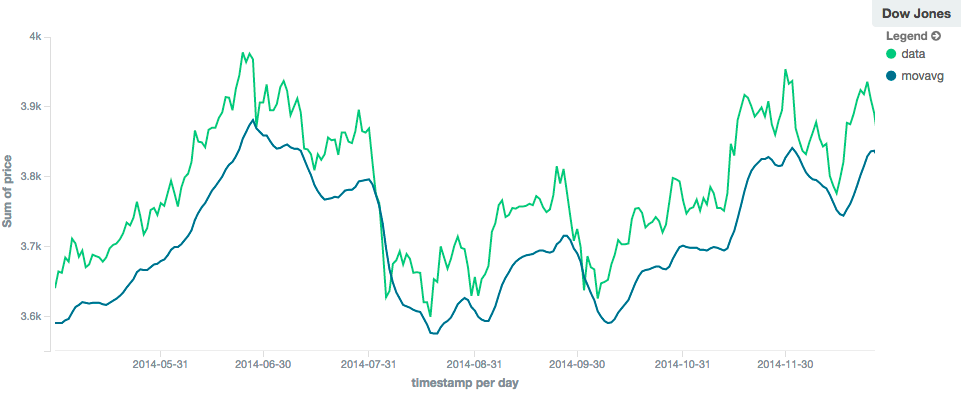Figure 3. Linear moving average with window of size 10

In contrast, a `linear` moving average with larger window (`"window": 100`) will smooth out all higher-frequency fluctuations, leaving only low-frequency, long term trends. It also tends to "lag" behind the actual data by a substantial amount, although typically less than the `simple` model: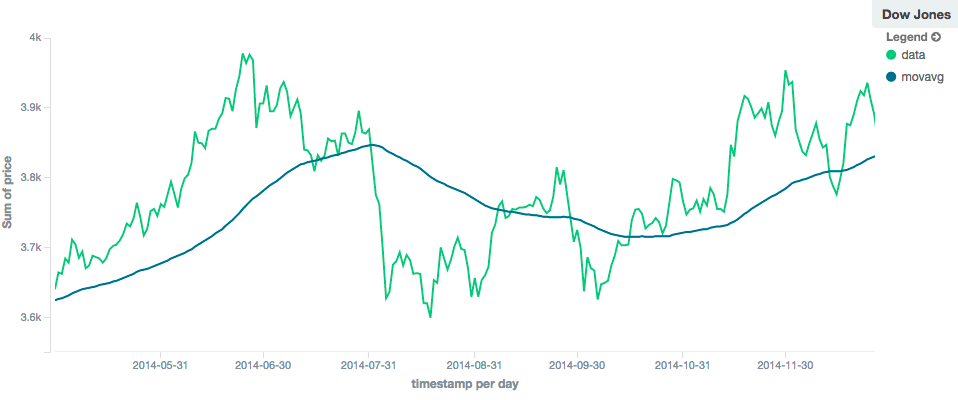Figure 4. Linear moving average with window of size 100

### EWMA (Exponentially Weighted)edit

The `ewma` model (aka "single-exponential") is similar to the `linear` model, except older data-points become exponentially less important, rather than linearly less important. The speed at which the importance decays can be controlled with an `alpha` setting. Small values make the weight decay slowly, which provides greater smoothing and takes into account a larger portion of the window. Larger values make the weight decay quickly, which reduces the impact of older values on the moving average. This tends to make the moving average track the data more closely but with less smoothing.

The default value of `alpha` is `0.3`, and the setting accepts any float from 0-1 inclusive.

The EWMA model can be Minimized

```POST /_search
{
"size": 0,
"aggs": {
"my_date_histo":{
"date_histogram":{
"field":"date",
"calendar_interval":"1M"
},
"aggs":{
"the_sum":{
"sum":{ "field": "price" }
},
"the_movavg": {
"moving_avg":{
"buckets_path": "the_sum",
"window" : 30,
"model" : "ewma",
"settings" : {
"alpha" : 0.5
}
}
}
}
}
}
}```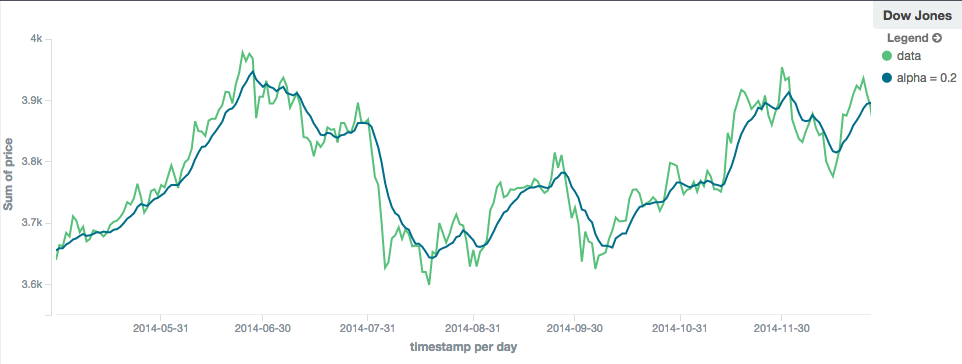Figure 5. EWMA with window of size 10, alpha = 0.2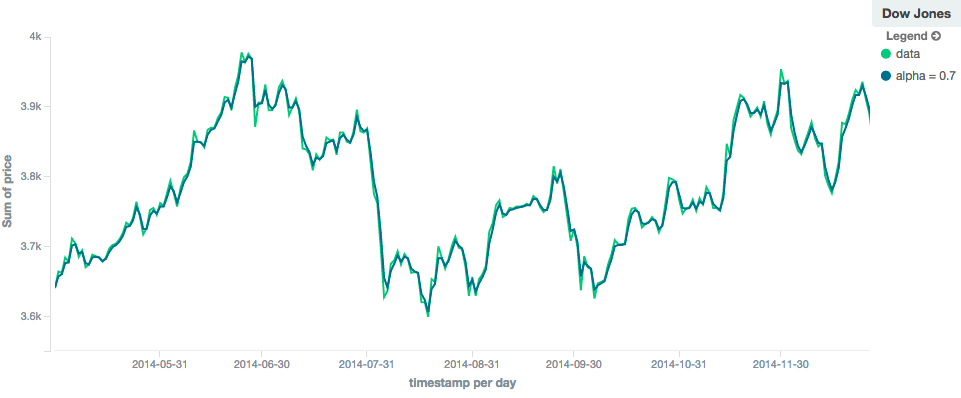Figure 6. EWMA with window of size 10, alpha = 0.7

### Holt-Linearedit

The `holt` model (aka "double exponential") incorporates a second exponential term which tracks the data’s trend. Single exponential does not perform well when the data has an underlying linear trend. The double exponential model calculates two values internally: a "level" and a "trend".

The level calculation is similar to `ewma`, and is an exponentially weighted view of the data. The difference is that the previously smoothed value is used instead of the raw value, which allows it to stay close to the original series. The trend calculation looks at the difference between the current and last value (e.g. the slope, or trend, of the smoothed data). The trend value is also exponentially weighted.

Values are produced by multiplying the level and trend components.

The default value of `alpha` is `0.3` and `beta` is `0.1`. The settings accept any float from 0-1 inclusive.

The Holt-Linear model can be Minimized

```POST /_search
{
"size": 0,
"aggs": {
"my_date_histo":{
"date_histogram":{
"field":"date",
"calendar_interval":"1M"
},
"aggs":{
"the_sum":{
"sum":{ "field": "price" }
},
"the_movavg": {
"moving_avg":{
"buckets_path": "the_sum",
"window" : 30,
"model" : "holt",
"settings" : {
"alpha" : 0.5,
"beta" : 0.5
}
}
}
}
}
}
}```

In practice, the `alpha` value behaves very similarly in `holt` as `ewma`: small values produce more smoothing and more lag, while larger values produce closer tracking and less lag. The value of `beta` is often difficult to see. Small values emphasize long-term trends (such as a constant linear trend in the whole series), while larger values emphasize short-term trends. This will become more apparently when you are predicting values.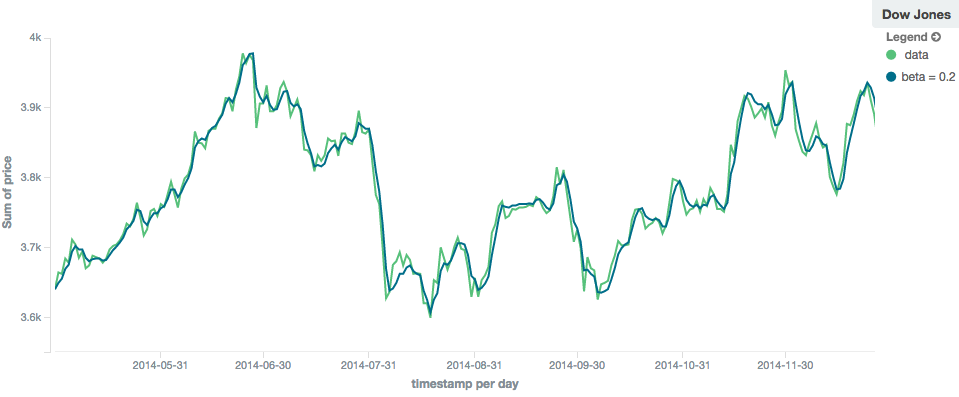Figure 7. Holt-Linear moving average with window of size 100, alpha = 0.5, beta = 0.2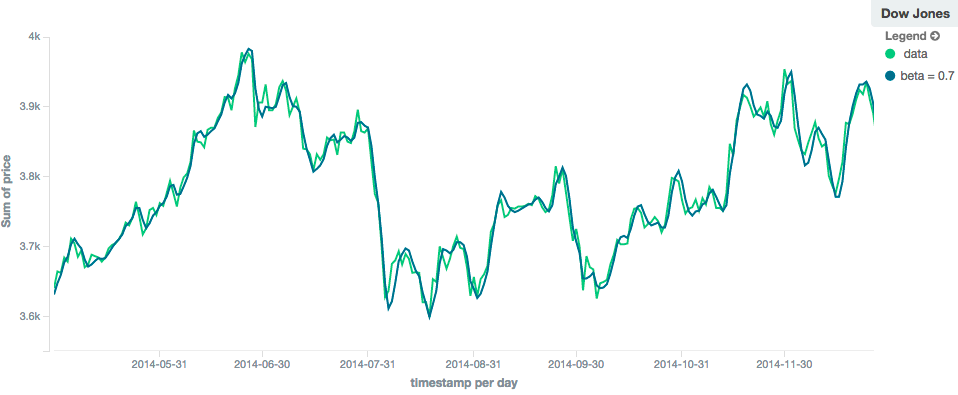Figure 8. Holt-Linear moving average with window of size 100, alpha = 0.5, beta = 0.7

### Holt-Wintersedit

The `holt_winters` model (aka "triple exponential") incorporates a third exponential term which tracks the seasonal aspect of your data. This aggregation therefore smooths based on three components: "level", "trend" and "seasonality".

The level and trend calculation is identical to `holt` The seasonal calculation looks at the difference between the current point, and the point one period earlier.

Holt-Winters requires a little more handholding than the other moving averages. You need to specify the "periodicity" of your data: e.g. if your data has cyclic trends every 7 days, you would set `period: 7`. Similarly if there was a monthly trend, you would set it to `30`. There is currently no periodicity detection, although that is planned for future enhancements.

There are two varieties of Holt-Winters: additive and multiplicative.

#### "Cold Start"edit

Unfortunately, due to the nature of Holt-Winters, it requires two periods of data to "bootstrap" the algorithm. This means that your `window` must always be at least twice the size of your period. An exception will be thrown if it isn’t. It also means that Holt-Winters will not emit a value for the first `2 * period` buckets; the current algorithm does not backcast.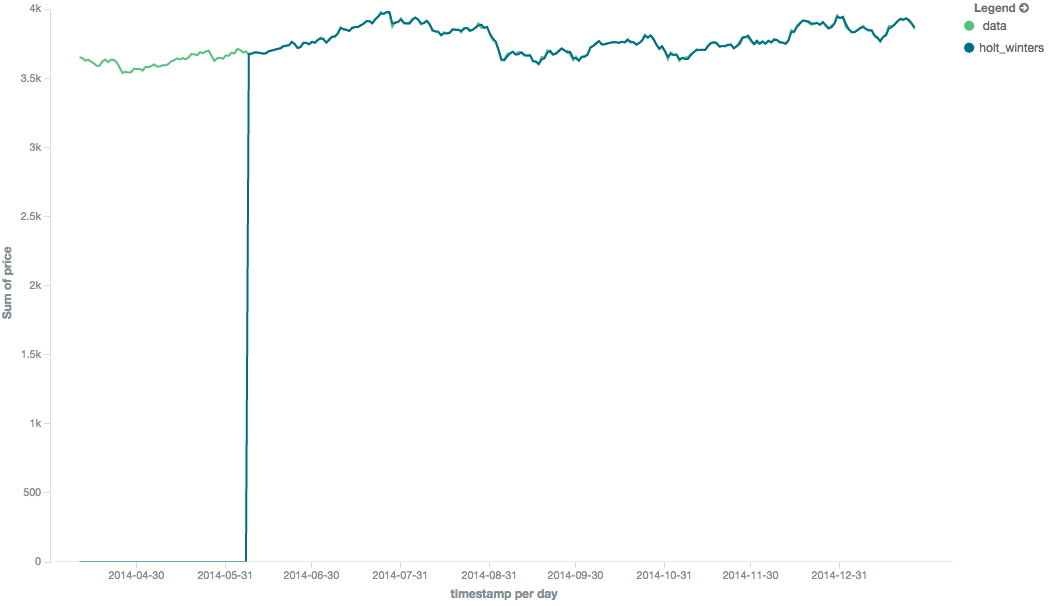Figure 9. Holt-Winters showing a "cold" start where no values are emitted

Because the "cold start" obscures what the moving average looks like, the rest of the Holt-Winters images are truncated to not show the "cold start". Just be aware this will always be present at the beginning of your moving averages!

Additive seasonality is the default; it can also be specified by setting `"type": "add"`. This variety is preferred when the seasonal affect is additive to your data. E.g. you could simply subtract the seasonal effect to "de-seasonalize" your data into a flat trend.

The default values of `alpha` and `gamma` are `0.3` while `beta` is `0.1`. The settings accept any float from 0-1 inclusive. The default value of `period` is `1`.

The additive Holt-Winters model can be Minimized

```POST /_search
{
"size": 0,
"aggs": {
"my_date_histo":{
"date_histogram":{
"field":"date",
"calendar_interval":"1M"
},
"aggs":{
"the_sum":{
"sum":{ "field": "price" }
},
"the_movavg": {
"moving_avg":{
"buckets_path": "the_sum",
"window" : 30,
"model" : "holt_winters",
"settings" : {
"alpha" : 0.5,
"beta" : 0.5,
"gamma" : 0.5,
"period" : 7
}
}
}
}
}
}
}```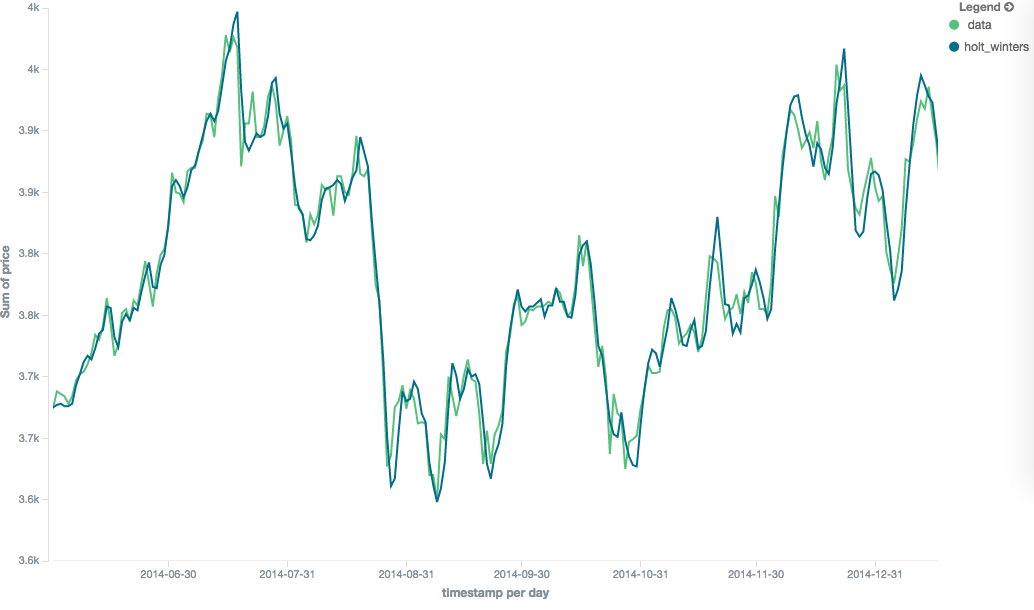Figure 10. Holt-Winters moving average with window of size 120, alpha = 0.5, beta = 0.7, gamma = 0.3, period = 30

#### Multiplicative Holt-Wintersedit

Multiplicative is specified by setting `"type": "mult"`. This variety is preferred when the seasonal affect is multiplied against your data. E.g. if the seasonal affect is x5 the data, rather than simply adding to it.

The default values of `alpha` and `gamma` are `0.3` while `beta` is `0.1`. The settings accept any float from 0-1 inclusive. The default value of `period` is `1`.

The multiplicative Holt-Winters model can be Minimized

Multiplicative Holt-Winters works by dividing each data point by the seasonal value. This is problematic if any of your data is zero, or if there are gaps in the data (since this results in a divid-by-zero). To combat this, the `mult` Holt-Winters pads all values by a very small amount (1*10-10) so that all values are non-zero. This affects the result, but only minimally. If your data is non-zero, or you prefer to see `NaN` when zero’s are encountered, you can disable this behavior with `pad: false`

```POST /_search
{
"size": 0,
"aggs": {
"my_date_histo":{
"date_histogram":{
"field":"date",
"calendar_interval":"1M"
},
"aggs":{
"the_sum":{
"sum":{ "field": "price" }
},
"the_movavg": {
"moving_avg":{
"buckets_path": "the_sum",
"window" : 30,
"model" : "holt_winters",
"settings" : {
"type" : "mult",
"alpha" : 0.5,
"beta" : 0.5,
"gamma" : 0.5,
"period" : 7,
}
}
}
}
}
}
}```

### Predictionedit

This functionality is experimental and may be changed or removed completely in a future release. Elastic will take a best effort approach to fix any issues, but experimental features are not subject to the support SLA of official GA features.

All the moving average model support a "prediction" mode, which will attempt to extrapolate into the future given the current smoothed, moving average. Depending on the model and parameter, these predictions may or may not be accurate.

Predictions are enabled by adding a `predict` parameter to any moving average aggregation, specifying the number of predictions you would like appended to the end of the series. These predictions will be spaced out at the same interval as your buckets:

```POST /_search
{
"size": 0,
"aggs": {
"my_date_histo":{
"date_histogram":{
"field":"date",
"calendar_interval":"1M"
},
"aggs":{
"the_sum":{
"sum":{ "field": "price" }
},
"the_movavg": {
"moving_avg":{
"buckets_path": "the_sum",
"window" : 30,
"model" : "simple",
"predict" : 10
}
}
}
}
}
}```

The `simple`, `linear` and `ewma` models all produce "flat" predictions: they essentially converge on the mean of the last value in the series, producing a flat: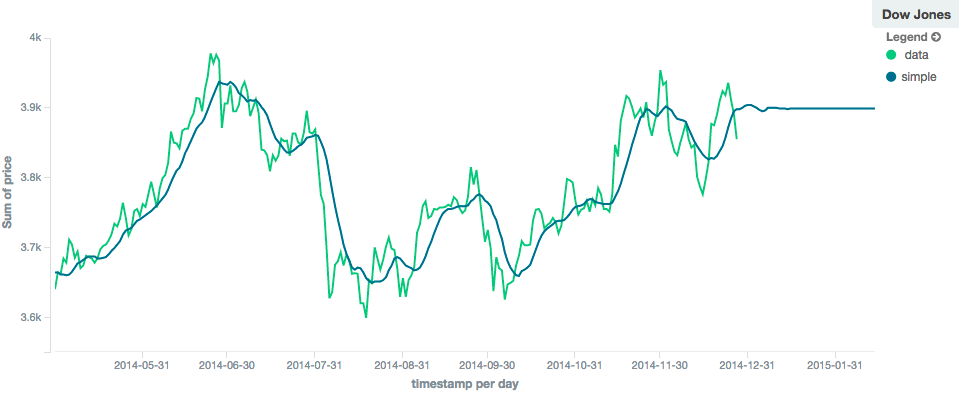Figure 11. Simple moving average with window of size 10, predict = 50

In contrast, the `holt` model can extrapolate based on local or global constant trends. If we set a high `beta` value, we can extrapolate based on local constant trends (in this case the predictions head down, because the data at the end of the series was heading in a downward direction):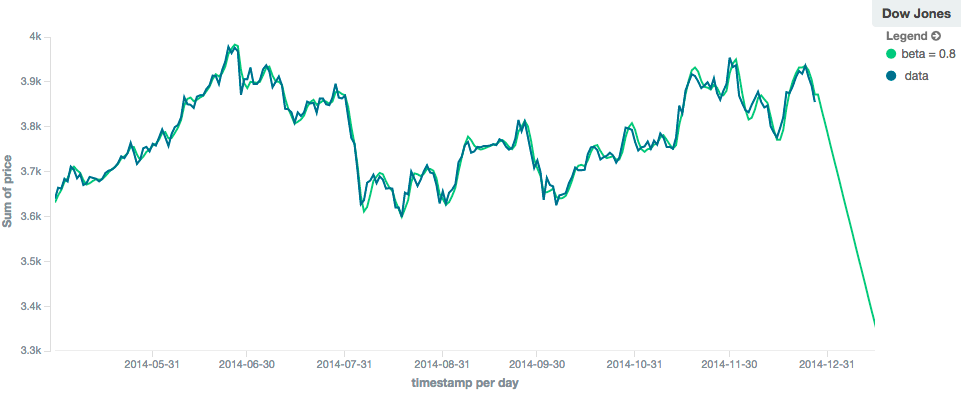Figure 12. Holt-Linear moving average with window of size 100, predict = 20, alpha = 0.5, beta = 0.8

In contrast, if we choose a small `beta`, the predictions are based on the global constant trend. In this series, the global trend is slightly positive, so the prediction makes a sharp u-turn and begins a positive slope: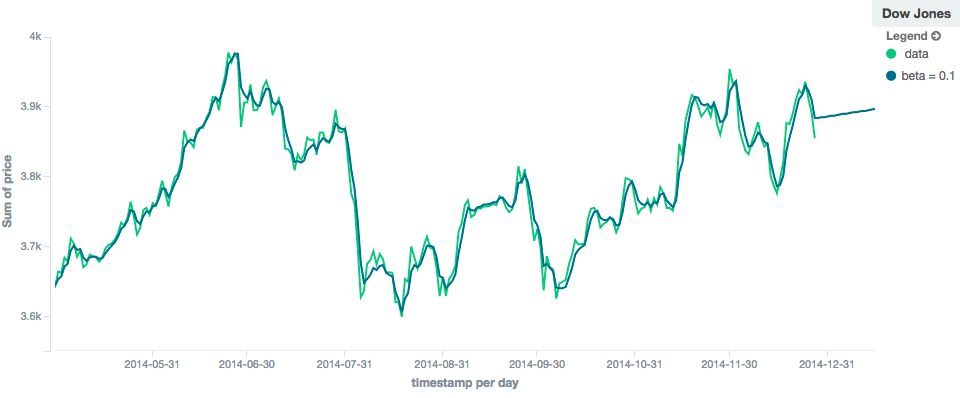Figure 13. Double Exponential moving average with window of size 100, predict = 20, alpha = 0.5, beta = 0.1

The `holt_winters` model has the potential to deliver the best predictions, since it also incorporates seasonal fluctuations into the model: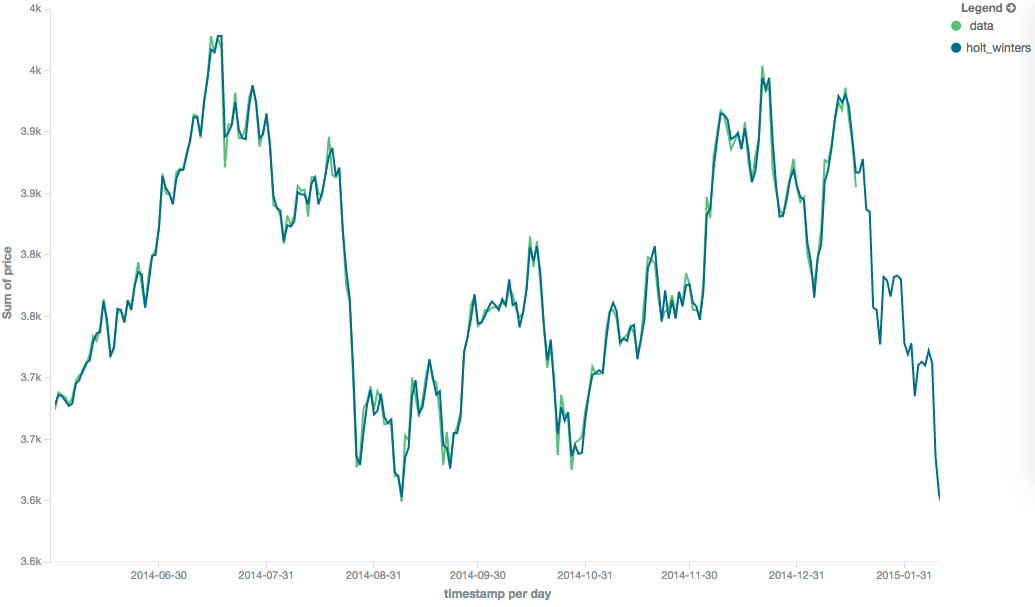Figure 14. Holt-Winters moving average with window of size 120, predict = 25, alpha = 0.8, beta = 0.2, gamma = 0.7, period = 30

### Minimizationedit

Some of the models (EWMA, Holt-Linear, Holt-Winters) require one or more parameters to be configured. Parameter choice can be tricky and sometimes non-intuitive. Furthermore, small deviations in these parameters can sometimes have a drastic effect on the output moving average.

For that reason, the three "tunable" models can be algorithmically minimized. Minimization is a process where parameters are tweaked until the predictions generated by the model closely match the output data. Minimization is not fullproof and can be susceptible to overfitting, but it often gives better results than hand-tuning.

Minimization is disabled by default for `ewma` and `holt_linear`, while it is enabled by default for `holt_winters`. Minimization is most useful with Holt-Winters, since it helps improve the accuracy of the predictions. EWMA and Holt-Linear are not great predictors, and mostly used for smoothing data, so minimization is less useful on those models.

Minimization is enabled/disabled via the `minimize` parameter:

```POST /_search
{
"size": 0,
"aggs": {
"my_date_histo":{
"date_histogram":{
"field":"date",
"calendar_interval":"1M"
},
"aggs":{
"the_sum":{
"sum":{ "field": "price" }
},
"the_movavg": {
"moving_avg":{
"buckets_path": "the_sum",
"model" : "holt_winters",
"window" : 30,
"minimize" : true,
"settings" : {
"period" : 7
}
}
}
}
}
}
}```
 Minimization is enabled with the `minimize` parameter

When enabled, minimization will find the optimal values for `alpha`, `beta` and `gamma`. The user should still provide appropriate values for `window`, `period` and `type`.

Minimization works by running a stochastic process called simulated annealing. This process will usually generate a good solution, but is not guaranteed to find the global optimum. It also requires some amount of additional computational power, since the model needs to be re-run multiple times as the values are tweaked. The run-time of minimization is linear to the size of the window being processed: excessively large windows may cause latency.

Finally, minimization fits the model to the last `n` values, where `n = window`. This generally produces better forecasts into the future, since the parameters are tuned around the end of the series. It can, however, generate poorer fitting moving averages at the beginning of the series.